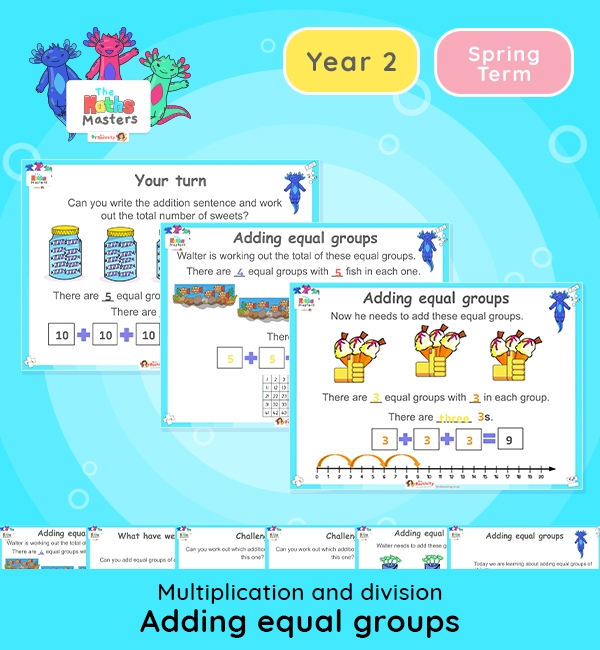# Year 2 | Adding Equal Groups Lesson Presentation## White Rose Year 2 multiplication and division lesson plans

Aligned with the White Rose Maths scheme of work, this Year 2 | Adding Equal Groups Lesson Presentation is fully editable, and is designed for the Year 2 maths curriculum covering the following maths objectives for the autumn term:

Topic: Multiplication and division

This lesson practises adding equal groups of 2s, 5s, 10s and 3s using repeated addition. Children also have learnt how to add three 1-digit numbers, so they can also add up to three equal groups of up to 9 objects.

It builds on learning of adding equal groups from the end of the autumn term.

White Rose Maths Small Steps: Add equal groups.

NC Links: Recall and use multiplication and division facts for the 2,5, and 10 times tables. *solve problems involving multiplication and division using materials, array, repeated addition, mental methods and multiplication and division facts including problems in context. *Count in steps of 2, 3, 5, and 10.

TAF Statements: Working At  – Recall and use multiplication and division facts for 2, 5, and 10 and use them to solve simple problems.

Greater Depth – Use reasoning about number and relationships to solve more complex problems and explain their thinking.

Year 1 conceptual prerequisites: Count in multiples of 2, 5 and 10

## Recently Viewed